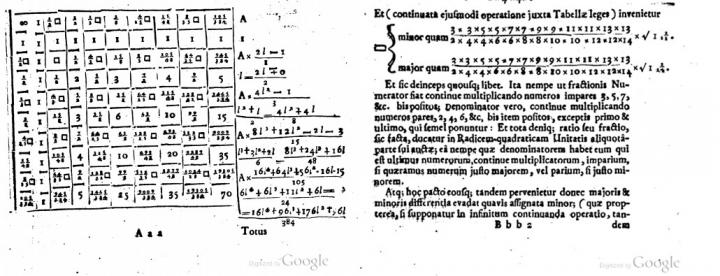News Release

# New derivation of pi links quantum physics and pure math

Researchers stumbled upon a famous pre-Newtonian formula for pi while computing the energy levels of a hydrogen atom

Peer-Reviewed Publication

American Institute of Physicsimage: Two pages from the book "Arithmetica Infinitorum," by John Wallis. In the table on the left page, the square that appears repeatedly denotes 4/pi, or the ratio of the area of a square to the area of the circumscribed circle. Wallis used the table to obtain the inequalities shown at the top of the page on the right that led to his formula. view more  Credit: Digitized by Google

WASHINGTON, D.C., November 10, 2015 - In 1655 the English mathematician John Wallis published a book in which he derived a formula for pi as the product of an infinite series of ratios. Now researchers from the University of Rochester, in a surprise discovery, have found the same formula in quantum mechanical calculations of the energy levels of a hydrogen atom.

"We weren't looking for the Wallis formula for pi. It just fell into our laps," said Carl Hagen, a particle physicist at the University of Rochester. Having noticed an intriguing trend in the solutions to a problem set he had developed for students in a class on quantum mechanics, Hagen recruited mathematician Tamar Friedmann and they realized this trend was in fact a manifestation of the Wallis formula for pi.

"It was a complete surprise - I jumped up and down when we got the Wallis formula out of equations for the hydrogen atom," said Friedmann. "The special thing is that it brings out a beautiful connection between physics and math. I find it fascinating that a purely mathematical formula from the 17th century characterizes a physical system that was discovered 300 years later."

The researchers report their findings in the Journal of Mathematical Physics, from AIP Publishing.

In quantum mechanics, a technique called the variational approach can be used to approximate the energy states of quantum systems, like molecules, that can't be solved exactly. Hagen was teaching the technique to his students when he decided to apply it to a real-world object: the hydrogen atom. The hydrogen atom is actually one of the rare quantum mechanical systems whose energy levels can be solved exactly, but by applying the variational approach and then comparing the result to the exact solution, students could calculate the error in the approximation.

When Hagen started solving the problem himself, he immediately noticed a trend. The error of the variational approach was about 15 percent for the ground state of hydrogen, 10 percent for the first excited state, and kept getting smaller as the excited states grew larger. This was unusual, since the variational approach normally only gives good approximations for the lowest energy levels.

Hagen recruited Friedmann to take a look at what would happen with increasing energy. They found that the limit of the variational solution approaches the model of hydrogen developed by physicist Niels Bohr in the early 20th century, which depicts the orbits of the electron as perfectly circular. This would be expected from Bohr's correspondence principle, which states that for large radius orbits, the behavior of quantum systems can be described by classical physics.

"At the lower energy orbits, the path of the electron is fuzzy and spread out," Hagen explained. "At more excited states, the orbits become more sharply defined and the uncertainty in the radius decreases."

From the formula for the limit of the variational solution as the energy increased, Hagen and Friedmann were able to pull out the Wallis formula for pi.

The theory of quantum mechanics dates back to the early 20th century and the Wallis formula has been around for hundreds of years, but the connection between the two had remained hidden until now.

"Nature had kept this secret for the last 80 years," Friedmann said. "I'm glad we revealed it."

###

The article, "Quantum mechanical derivation of the Wallis formula for pi," is authored by Tamar Friedmann and C.R. Hagen. It will be published in the Journal of Mathematical Physics on November 10, 2015 (DOI: 10.1063/1.4930800). After that date, it can be accessed at: http://scitation.aip.org/content/aip/journal/jmp/56/11/10.1063/1.4930800

The authors of this paper are affiliated with the University of Rochester.